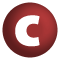# Reactions

#### class Reactions extends PartialFunction[Event, Unit]

Used by reactors to let clients register custom event reactions.

attributes: abstract
known subclasses: Impl

### Inherits

1. PartialFunction
2. Function1
3. AnyRef
4. Any

### Value Members

1. #### def +=(r: PartialFunction[Event, Unit]): Reactions

attributes: abstract
2. #### def -=(r: PartialFunction[Event, Unit]): Reactions

Remove the given reaction

Remove the given reaction.

attributes: abstract
3. #### def andThen[C](k: (Unit) ⇒ C): PartialFunction[Event, C]

Composes this partial function with a transformation function that gets applied to results of this partial function

Composes this partial function with a transformation function that gets applied to results of this partial function.

C

the result type of the transformation function.

k

the transformation function

returns

a partial function with the same domain as this partial function, which maps arguments `x` to `k(this(x))`.

definition classes: PartialFunctionFunction1

5. #### def compose[A](g: (A) ⇒ Event): (A) ⇒ Unit

(f compose g)(x) == f(g(x))

(f compose g)(x) == f(g(x))

definition classes: Function1
6. #### def equals(arg0: Any): Boolean

This method is used to compare the receiver object (`this`) with the argument object (`arg0`) for equivalence

This method is used to compare the receiver object (`this`) with the argument object (`arg0`) for equivalence.

The default implementations of this method is an equivalence relation:

• It is reflexive: for any instance `x` of type `Any`, `x.equals(x)` should return `true`.
• It is symmetric: for any instances `x` and `y` of type `Any`, `x.equals(y)` should return `true` if and only if `y.equals(x)` returns `true`.
• It is transitive: for any instances `x`, `y`, and `z` of type `AnyRef` if `x.equals(y)` returns `true` and `y.equals(z)` returns `true`, then `x.equals(z)` should return `true`.

If you override this method, you should verify that your implementation remains an equivalence relation. Additionally, when overriding this method it is often necessary to override `hashCode` to ensure that objects that are "equal" (`o1.equals(o2)` returns `true`) hash to the same `Int` (`o1.hashCode.equals(o2.hashCode)`).

arg0

the object to compare against this object for equality.

returns

`true` if the receiver object is equivalent to the argument; `false` otherwise.

definition classes: AnyRef ⇐ Any
7. #### def hashCode(): Int

Returns a hash code value for the object

Returns a hash code value for the object.

The default hashing algorithm is platform dependent.

Note that it is allowed for two objects to have identical hash codes (`o1.hashCode.equals(o2.hashCode)`) yet not be equal (`o1.equals(o2)` returns `false`). A degenerate implementation could always return `0`. However, it is required that if two objects are equal (`o1.equals(o2)` returns `true`) that they have identical hash codes (`o1.hashCode.equals(o2.hashCode)`). Therefore, when overriding this method, be sure to verify that the behavior is consistent with the `equals` method.

definition classes: AnyRef ⇐ Any
8. #### def isDefinedAt(x: Event): Boolean

Checks if a value is contained in the function's domain

Checks if a value is contained in the function's domain.

x

the value to test

returns

`true`, iff `x` is in the domain of this function, `false` otherwise.

attributes: abstract
definition classes: PartialFunction
9. #### def lift: (Event) ⇒ Option[Unit]

Turns this partial function into an plain function returning an `Option` result

Turns this partial function into an plain function returning an `Option` result.

definition classes: PartialFunction
10. #### def orElse[A1 <: Event, B1 >: Unit](that: PartialFunction[A1, B1]): PartialFunction[A1, B1]

Composes this partial function with a fallback partial function which gets applied where this partial function is not defined

Composes this partial function with a fallback partial function which gets applied where this partial function is not defined.

A1

the argument type of the fallback function

B1

the result type of the fallback function

that

the fallback function

returns

a partial function which has as domain the union of the domains of this partial function and `that`. The resulting partial function takes `x` to `this(x)` where `this` is defined, and to `that(x)` where it is not.

definition classes: PartialFunction
11. #### def toString(): String

Returns a string representation of the object

Returns a string representation of the object.

The default representation is platform dependent.

definition classes: Function1 ⇐ AnyRef ⇐ Any